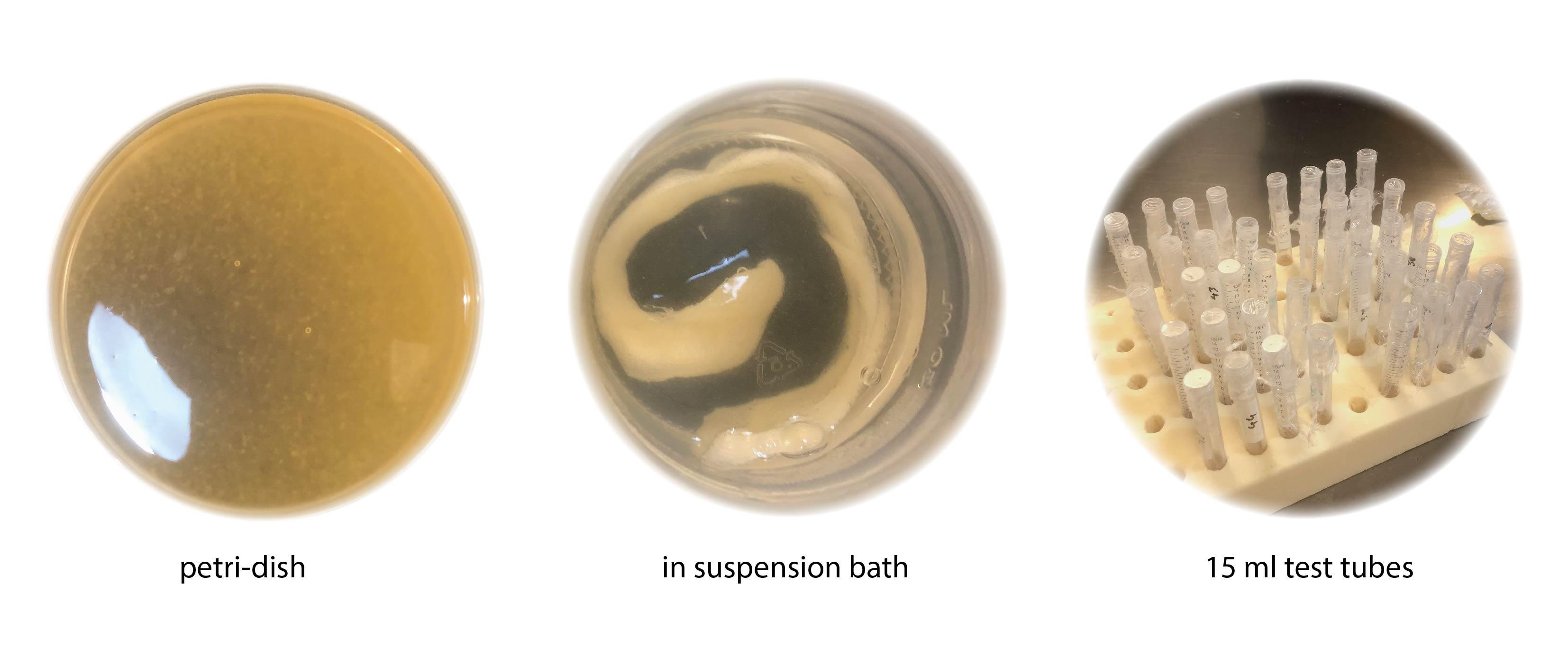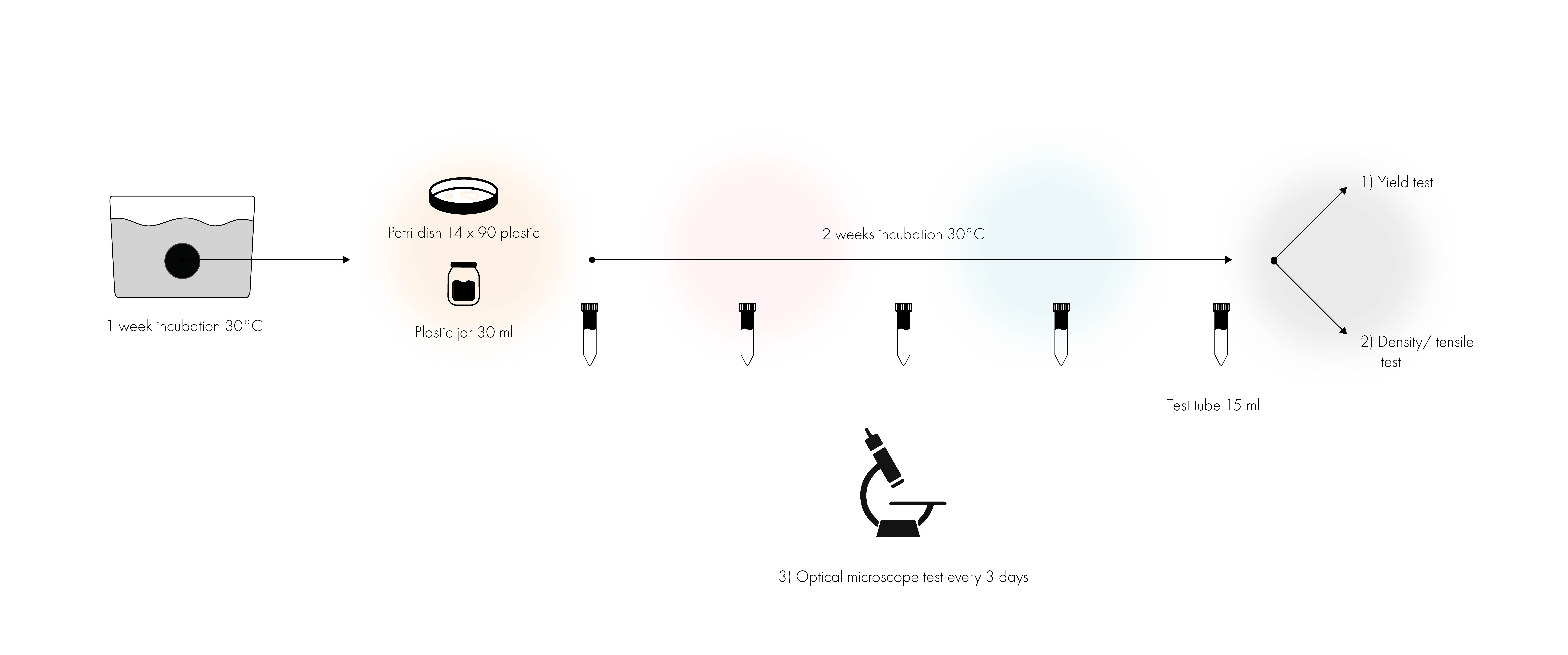# 06 Methodology

### Problem 3: Analyses¶

To be able to analyse my experiment in a simple but reliable way without many of the fancy equipment’s often used I decided on different methods.

#### roadmap A and B for living-ink¶1) Yield in g/L- will give an idea of how different hydrogel recipes are effecting the growth speed of BC
2) Water loss in %– will give indication of how different hydrogel recipes will affect the quality and density of the BC
3) Visual microscopic tracking- For a deeper understanding of the visual development.1 and 2 I got following protocol from Clara Davis thesis:

“ the calculation of the yield in g/L and the water loss in %. The first provides information on the quantity of cellulose produced, the second gives an idea of the quality. The production yield of BNC can be defined as the productivity and capacity of bacteria to secrete cellulose. Concerning the loss of water, the hypothesis is that the more the membrane loses liquid, the greater the rearrangement of internal structure in the polymer matrix.
Moreover, if less water is lost, the denser the inter- and intra-molecular hydrogen bonds of cellulose, and the higher the degree of crystallinity. Therefore, a BNC membrane with a high-water loss percentage will theoretically have a lower tensile strength.” C. Davis

Yield:The production yield of the samples was obtained gravimetrically by using the dry weight (𝑊 ) 𝑑 in (g) of the collected membrane and the initial volume of the culture medium (𝑉 ) in (L). BNC yield 𝑖 was calculated according to the following equation:

BNC is a highly porous fibrillar network that is synthesized as a gel-like membrane in a liquid medium. In its never-dried state (see Figure 1-22), the BNC matrix can hold a tremendous amount of water because most molecular hydrogen-bonds are not yet formed. Calculating the initial water loss from the BNC provides information on the density and quality of the fibrillar structure. Water loss from BNC can be determined using the following formula:

density and tensile strengthWhere 𝐵𝑁𝐶 𝑊 is the wet weight of BNC (never-dried state) and 𝐵𝑁𝐶 𝑊 is the dry weight of BNC 𝑤𝑑 after first drying.

3 to have a more visual understanding of the growth development I decided to track the development of the test during every 3 days by placing 2ml of the each culture medium in test tubes covered with perforated plastic wrap

1. Prepare a safranin staining solution by mixing 1 g of safranin in 100 ml of distilled water.
2. Strain the hydrogel samples with the safranin solution and incubate for 10 minutes.
3. Heat fixate the samples
4. Wash the samples with distilled water and gently blot them to remove excess solution.
5. Observe the samples under an optical microscope using a bright-field mode.
6. Take photographs of the samples at different magnifications and use a stage micrometer to accurately measure the length and width of the bacterial cellulose in the hydrogel.
7. Calculate the growth of bacterial cellulose by measuring the change in the area of the samples from day 0 to day 12.
8. Repeat the process for multiple samples to increase the accuracy of the results.
9. Analyze the results and make conclusions about the growth of bacterial cellulose in the hydrogel over the 12 days.

Protocol summarized by ChatGBT

#### roadmap C for purified SCOBY¶

The weight was determined using a digital scale Mettler, model AE240, with an accuracy of 0.0001g. The dimensions were taken with a millimeter ruler and the thickness with a gauge micrometer SHAHE with a precision of 0.01mm. The measurements of the samples are listed in the Annexes. With these measurements, different data could be approximated and calculated such as the density 𝑑 and the grammage 𝐺 (surface density) of the samples. The density and grammage were calculated using the following equations:Where 𝑚 the masse (g), 𝑣 the volume (mm3), 𝑙 the length (mm), 𝑤 the width (mm), 𝑡 the thickness (mm), and 𝐴 the surface area (m2) of the sample.

here is excel with all raw data and calculations

Last update: 2023-04-20